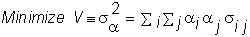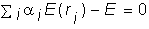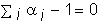4.7 THE MARKOWITZ PROBLEM WITH SHORT SALES

Solving the portfolio choice problem requires the use of calculus and matrix manipulation.

Formally, the objective of the mathematical problem is to minimize portfolio risk subject to a target return constraint, plus the wealth allocation constraint that all wealth is fully invested in the portfolio. From the definition of portfolio risk, provided in Chapter 2, the portfolio problem formally issubject to the two constraints:The first constraint above fixes some target expected rate of return, E. The second constrains the portfolio weights to sum to one (with no restrictions on short selling). Note that the solution to this problem satisfies dominance because it minimizes variance for a given expected return.

You can see how this problem is formally solved in topic 4.8, Solution to Markowitz Problem with Short Sales. An application of the solution is provided in topic 4.9, Three-Firm Case: Application of Mean Variance Theory.

previous topic

next topic